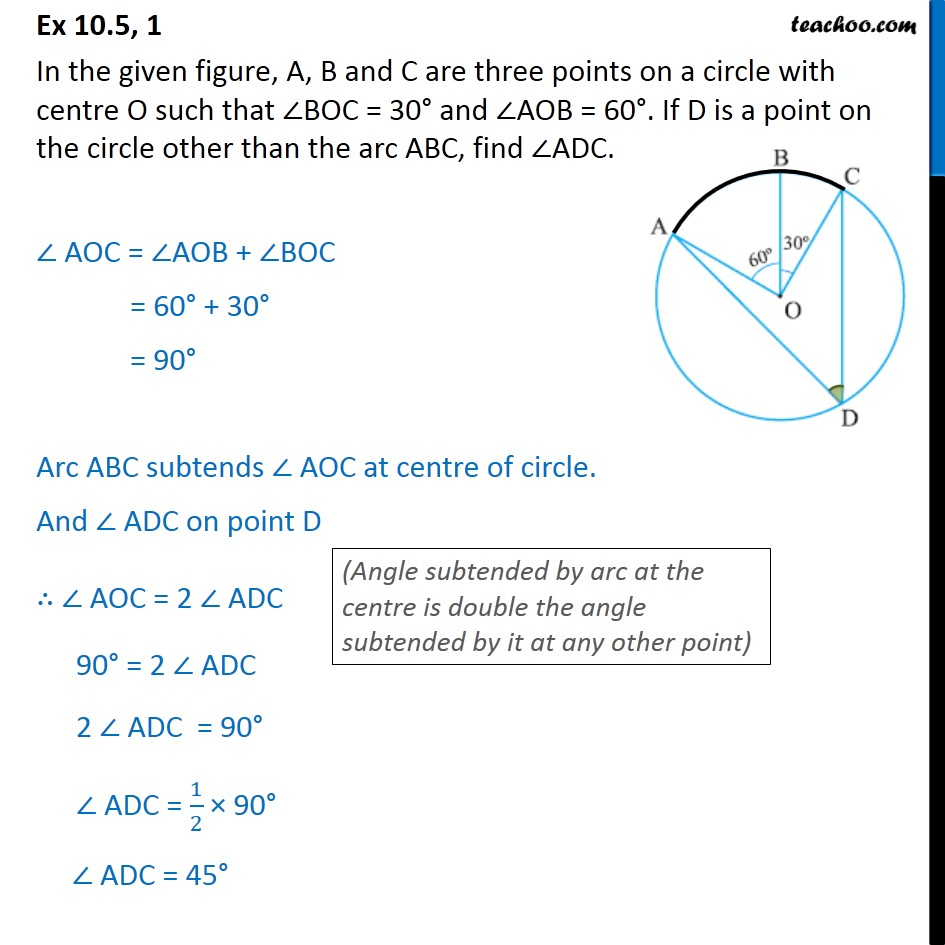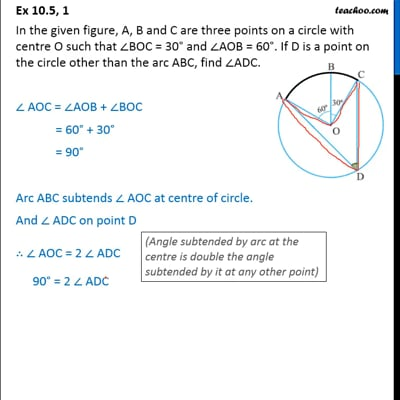Ex 9.3

Chapter 9 Class 9 Circles
Serial order wiseThis video is only available for Teachoo black users

Learn in your speed, with individual attention - Teachoo Maths 1-on-1 Class

### Transcript

Ex 9.3, 1 In the given figure, A, B and C are three points on a circle with centre O such that BOC = 30 and AOB = 60 . If D is a point on the circle other than the arc ABC, find ADC. AOC = AOB + BOC = 60 + 30 = 90 Arc ABC subtends AOC at centre of circle. And ADC on point D AOC = 2 ADC 90 = 2 ADC 2 ADC = 90 ADC = 1/2 90 ADC = 45